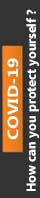We're Open

0% Plagiarism Guaranteed & Custom Written

# 1. A cover page giving your name and your student number and including the signed statement

EG-264 - MATLAB Coursework

Basic details

You are asked to submit your MATLAB solution to the problems specified below. A professional presentation of your solution is required. This should contain:

1. A cover page giving your name and your student number and including the signed statement "I confirm that I have not received help from, or given help to, anyone else in constructing the solution to this assignment".

2. A brief description of the problem and of the process you have followed to produce your solution.

3. A complete documented listing of your actual MATLAB files (i.e. NOT retyped by you and NO screenshots).

4. A listing of the actual output from MATLAB giving the answers requested (i.e. NOT retyped by you and NO screenshots).

5. Appropriate referencing to any publication or web based material that you have used in constructing your solution.

Question 1: Figure 1 shows the speed (mph) against time(s) graph of a Camaro car accelerating from a standing start. Over 25 seconds the car accelerates to nearly 150mph. Figure 1: Speed (mph) vs. Time(s) graph of a 2010 Camaro’s data measurements. To reproduce the speed and time data from the image, combine equations (1), (2) and (3) using for loops and conditional statements. When t < 5 s, the speed of the car in mph can be determined using Equation (1): Speed(t) = 0.1553567(t6 ) - 2.0416(t5 ) + 9.1837(t4 ) – 14.829(t3 ) - 1.3703(t2 ) + 32.821(t) - 1.3155 (Eqn. 1) When 𝟓𝒔 ≤ 𝒕 < 𝟏𝟓. 𝟒𝒔, the speed of the car in mph can be determined using Equation (2): Speed(t) = 0.003980879(t5 ) – 0.2247(t4 ) + 4.8682(t3 ) – 50.442(t2 ) + 254.67(t) - 430.66 (Eqn. 2) When t ≥ 15.4s, the speed of the car in mph can be determined using Equation (3): Speed(t) = -0.073(t2 ) + 6.1802(t) + 40.423 (Eqn. 3)

To determine the distance travelled by the Camaro, numerical integration can be used on the reproduced speed vs. time data, calculating the area under the curve

Use the composite trapezoidal rule to obtain an approximation of the distance travelled by the Camaro in metres, with a relative error of less than 0.00002%. During the numerical integration calculations, if the relative error is not reached, double the number of separations used over the timespan in the calculations for the following calculation

(i) In the command window, display the integral value calculated for distance, the number of sections used in the numerical integration, and the relative error produced for each looped calculation using ‘fprintf’ and associated commands.

(ii) Produce a single figure with two subplots, (1) showing the speed (m/s) vs. time (t) of the combined speed equations in one plot at a reasonable accuracy, and (2) a cumulative distance (m) graph of the Camaro over time(s) in the second subplot.

(iii) Produce a figure showing the total distance calculated against the number of separations used in each numerical integration calculation; use a logarithmic x-axis scale on the resulting plot.

Question 2: An undamped system is harmonically excited using an external forcing frequency 𝜔, which produces vibration of the system. The displacement of the system over time (x(t)) can be calculated using Equation 4, when using the parameter values detailed in Table 1.

The acting driving frequency 𝜔 however is unknown.

(i) Use the bisection method to determine an approximation of the value of 𝜔, with an absolute error of less than 1 × 10−6 ;when the displacement x = 0.0386m, at time t = 4.54s, limiting the useable values of 𝜔 between: 5.12 < 𝜔 < 6.45. Display the resulting value of 𝜔, and the absolute error obtained.

(ii) Produce a plot of the displacement x(t), between 0 < 𝑡 < 6𝑠, using the driving frequency 𝜔 calculated in part (i).

100% Plagiarism Free & Custom Written,